• 时间复杂度和空间复杂度
2021-06-11 20:21:12

# 复杂度的含义和表示复杂度的符号

在数据结构和算法中，需要对时间复杂度和空间复杂度进行分析。时间复杂度用来表示算法运行时间和问题规模之间的渐近关系，空间复杂度用来表示算法所使用的空间和问题规模之间的渐进关系。

常见的表示复杂度的符号有三种： O O Ω \varOmega Θ \varTheta

• O O 表示复杂度的上界。
• Ω \varOmega 表示复杂度的下界。
• Θ \varTheta 表示复杂度的确界。

考虑一个问题：给定正整数 n n ，计算 ∑ i = 1 n i \sum\limits_{i=1}^n i

这个问题有两种方法可以解决。

• 方法一：使用循环计算，则需要进行 n n 次相加的操作，时间复杂度是 Θ ( n ) \varTheta(n)
• 方法二：使用等差数列求和公式计算，答案为 n ( n + 1 ) 2 \frac{n(n+1)}{2} ，时间复杂度是 Θ ( 1 ) \varTheta(1)

方法一的时间复杂度是 Θ ( n ) \varTheta(n) 。如果用 O O 表示，则可以表示成 O ( n ) O(n) ，也可以表示成 O ( n 2 ) O(n^2) O ( 2 n ) O(2^n) 。如果用 Ω \varOmega 表示，则可以表示成 Ω ( n ) \varOmega(n) ，也可以表示成 Ω ( log ⁡ n ) \varOmega(\log n) Ω ( 1 ) \varOmega(1)

实际应用中，最常用的符号是 O O ，且其含义和 Θ \varTheta 相同，即使用 O O 表示复杂度的确界。因此，在实际应用中，上述问题的方法一的时间复杂度应该表示成 O ( n ) O(n) ，而不应该表示成 O ( n 2 ) O(n^2) O ( 2 n ) O(2^n)

# 复杂度的表示的原则

复杂度的表示的原则是，只保留最高阶项，忽略低阶项和常数项。因为复杂度考虑的是当问题规模趋向于无穷大时，算法运行时间或使用空间和问题规模之间的渐近关系，最高阶项对复杂度的影响最大，所以只保留最高阶项。

例如，下列复杂度的表示都应该进行简化。

• O ( 2 n ) O(2n) 应简化成 O ( n ) O(n)
• O ( n ( n − 1 ) 2 ) O(\frac{n(n-1)}{2}) 应简化成 O ( n 2 ) O(n^2)
• O ( 100 × 2 n + 2020 n 2021 ) O(100 \times 2^n+2020n^{2021}) 应简化成 O ( 2 n ) O(2^n)

由于复杂度的表示只保留最高阶项，忽略低阶项和常数项，因此在数据规模较小的时候，可能会出现时间复杂度较低的算法用时反而更多的情况，这样的情况和复杂度分析的结果并不矛盾。

# 时间复杂度

时间复杂度表示算法运行时间和问题规模之间的渐近关系。常见的时间复杂度按照从低到高的顺序排列如下：

• 常数时间复杂度， O ( 1 ) O(1)
• 对数时间复杂度， O ( log ⁡ n ) O(\log n) （其中 log ⁡ \log 表示以 2 2 为底的对数）；
• 线性时间复杂度， O ( n ) O(n)
• 线性对数时间复杂度， O ( n log ⁡ n ) O(n\log n)
• 平方时间复杂度， O ( n 2 ) O(n^2)
• 立方时间复杂度， O ( n 3 ) O(n^3)
• 指数时间复杂度， O ( k n ) O(k^n) （其中 k k 为大于 1 1 的正整数常数）；
• 阶乘时间复杂度， O ( n ! ) O(n!)

算法的时间复杂度主要取决于算法中的循环和递归。一般而言，如果有 k k 层嵌套的循环，则时间复杂度是 O ( n k ) O(n^k) 。对于具体问题需要根据问题的实际情况进行具体分析，例如有时虽然有两层嵌套的循环，但是时间复杂度仍然可能是 O ( n ) O(n) 而不是 O ( n 2 ) O(n^2)

对于递归的情况，如果只有一个递归分支，则时间复杂度是线性的，如果产生多个分支且没有记忆化，则时间复杂度是指数级的。

# 空间复杂度

空间复杂度用来表示算法所使用的空间和问题规模之间的渐进关系。和时间复杂度相比，空间复杂度的分析相对简单。

空间复杂度主要取决于两个方面：

• 算法中的数据结构所使用的空间；
• 如果算法中有递归，则需要考虑递归调用栈的空间。

从复杂度分析的角度考虑，分析空间复杂度时，一般不考虑算法的输入参数和输出参数。

# 时间复杂度和空间复杂度的取舍

时间复杂度和空间复杂度经常是矛盾的。如果需要降低算法的时间复杂度，则通常需要增加算法的空间复杂度；如果想减少额外空间的使用，则通常会导致算法的时间复杂度增加。因此，如果需要对算法进行复杂度方面的优化，就有两个策略，一是优化时间复杂度，二是优化空间复杂度。

大多数情况下，时间复杂度的优化是更重要的，程序运行的时间是无法回收的，而空间是可以回收的，因此应该优先考虑降低时间复杂度。

只有在少数情况下，空间复杂度的优化更重要，例如计算资源有限，则不能使用过多的额外空间，因此需要降低空间复杂度。数据结构
更多相关内容
• 算法复杂度分为时间复杂度和空间复杂度。 其作用： 时间复杂度是指执行算法所需要的计算工作量； 而空间复杂度是指执行这个算法所需要的内存空间。 （算法的复杂性体现在运行该算法时的计算机所需资源的多少上，...
• 算法时间复杂度和空间复杂度 1.算法效率 算法效率分析分为两种：第一种是时间效率，第二种是空间效率。时间效率被称为时间复杂度，而空间效率被称作空间复杂度时间复杂度主要衡量的是一个算法的运行速度，而空间...

# 1.算法效率

算法效率分析分为两种：第一种是时间效率，第二种是空间效率。时间效率被称为时间复杂度，而空间效率被称作空间复杂度。 时间复杂度主要衡量的是一个算法的运行速度，而空间复杂度主要衡量一个算法所需要的额外空间，在计算机发展的早期，计算机的存储容量很小。所以对空间复杂度很是在乎。但是经过计算机行业的迅速发展，计算机的存储容量已经达到了很高的程度。所以我们如今已经不需要再特别关注一个算法的空间复杂度。

# 2.时间复杂度

2.1 时间复杂度的概念
时间复杂度的定义：在计算机科学中，算法的时间复杂度是一个
函数（数学中带有未知表达式的函数），它定量描述了该算法的运行时间。一个算法执行所耗费的时间，从理论上说，是不能算出来的，只有你把你的程序放在机器上跑起来，才能知道。但是我们需要每个算法都上机测试吗？是可以都上机测试，但是这很麻烦，所以才有了时间复杂度这个分析方式。一个算法所花费的时间与其中语句的执行次数成正比例，算法中的基本操作的执行次数，为算法的时间复杂度。

# 3.空间复杂度

空间复杂度是对一个算法在运行过程中临时占用存储空间大小量度 。空间复杂度不是程序占用了多少bytes的空间，因为这个也没太大意义，所以空间复杂度算的是变量的个数。空间复杂度计算规则基本跟实践复杂度类似，也使用大O渐进表示法。

# 4.大O渐进表示法

• 大O符号（Big O notation）：是用于描述函数渐进行为的数学符号。推导大O阶方法：
• 1、用常数1取代运行时间中的所有加法常数。
• 2、在修改后的运行次数函数中，只保留最高阶项。
• 3、如果最高阶项存在且不是1，则去除与这个项目相乘的常数。得到的结果就是大O阶。

另外有些算法的时间复杂度存在最好、平均和最坏情况：

• 最坏情况：任意输入规模的最大运行次数(上界) 平均情况：任意输入规模的期望运行次数
• 最好情况：任意输入规模的最小运行次数(下界) 例如：在一个长度为N数组中搜索一个数据x 最好情况：1次找到 最坏情况：N次找到
• 平均情况：N/2次找到 在实际中一般情况关注的是算法的最坏运行情况，所以数组中搜索数据时间复杂度为O(N)

# 5.常见时间复杂度

计算一下这个算法的时间复杂度

// 请计算一下Func1基本操作执行了多少次？void Func1(int N) { int count = 0;//两层循环嵌套外循环执行n次，内循环执行n次，整体计算就是N*N的执行次数for (int i = 0; i < N ; ++ i) { 	 for (int j = 0; j < N ; ++ j)	 { 		 ++count;	 }}//2 * N的执行次数for (int k = 0; k < 2 * N ; ++ k){ 	 ++count;}//常数项10int M = 10;while (M--) {  	++count;  } printf("%d\n", count);}


精确的时间复杂度是N ^ 2 + 2 * N + 10
大O的渐进表示法时间复杂度是O(N ^ 2)
分析：
1、两层循环嵌套外循环执行n次，内循环执行n次，整体计算就是N*N 的执行次数
2、2 * N的执行次数
3、常数项10
根据前面的大o渐进表示法规则，所以最后只保留那项对执行次数影响最大的那一项，时间复杂度就是O(N ^ 2)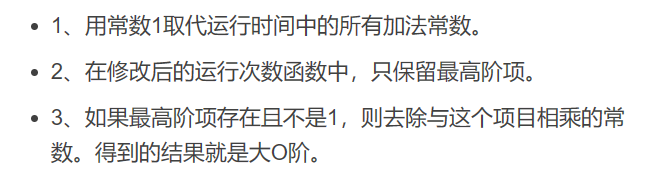计算一下这个算法的时间复杂度

// 计算Func2的时间复杂度？void Func2(int N) { 	int count = 0;	for (int k = 0; k < 2 * N ; ++ k) 	{ 		++count; 	}		int M = 10;	while (M--) 	{ 	++count; }	printf("%d\n", count);}


精确的算法执行次数是2 * n + 10
采用大O渐进表示法规则后时间复杂度是O(N)
如果最高阶项存在且不是1，则去除与这个项目相乘的常数，那保留的就会是O(N)

计算一下这个算法的时间复杂度

// 计算Func3的时间复杂度？void Func3(int N, int M){ 	//执行M次	int count = 0;	for (int k = 0; k < M; ++ k) 	{ 		++count; 	}	//执行N次	for (int k = 0; k < N ; ++ k) 	{ 		++count; 	}	printf("%d\n", count);}


时间复杂度：O(M + N)
假设：
M远大于N --> O(M)
N远大于M --> O(N)
M和N一样大 --> O(M) / O(N）
还是取影响最大的那一项，如果并没有说明M和N的大小关系，那么时间复杂度就是O(M + N)

计算一下这个算法的时间复杂度

// 计算Func4的时间复杂度？void Func4(int N) { 	int count = 0;	for (int k = 0; k < 100; ++ k) 	{ 		++count; 	}	printf("%d\n", count);}


时间复杂度：O(1)
循环执行的次数是常数次，常数取1

计算一下这个算法的时间复杂度

// 计算strchr的时间复杂度？const char * strchr ( const char * str, int character ){ 	while(*str)	{ 		if(*str == character)			return *str;		else			str++;	}}


时间复杂度：O(N)
最坏情况：遍历到最后才找到或者字符串中压根就没有，O(N)
平均情况：O(N / 2) ， 忽略系数项是O(N)
最好情况：一次就找到了，O(1)

计算一下这个算法的时间复杂度

// 计算BubbleSort的时间复杂度？void BubbleSort(int* a, int n) { 	assert(a);	for (size_t end = n; end > 0; --end)	 { 		int exchange = 0;		for (size_t i = 1; i < end; ++i)		{ 			if (a[i-1] > a[i])			{ 				Swap(&a[i-1], &a[i]);				exchange = 1;			}		}		if (exchange == 0)		break; 	} }


精确的时间复杂度：O(N * (N-1) / 2)
冒泡排序的思想是：假设给你N个元素，让你排升序，对N个元素排升序，只需要执行N - 1趟，两两相比较，每趟交换都会将最大的那个元素换到最后面去，这样子一来最大的数就都集中在后面，不就相当于每排序一趟后就少比较一个元素嘛，因为后面已经是最大的了不需要再交换。
通过公式展开后采用大O渐进表示法规则后，时间复杂度：O(N ^ 2)

计算一下这个算法的时间复杂度

// 计算BinarySearch的时间复杂度？int BinarySearch(int* a, int n, int x){ 	assert(a);	int begin = 0;	int end = n-1; .		while (begin < end) 	{ 		int mid = begin + ((end-begin)>>1);		if (a[mid] < x)			begin = mid+1;		else if (a[mid] > x)			end = mid;		else			return mid; 	}	return -1; }


先来理解二分查找的思想：
二分查找是通过下标来搜索对应的元素值，前提是这个数组是有序的，通过确定中间位置划分左右区间，如果val小于mid那么目标值排除了出现在右边的情况，继续再左边区间查找，确定中间下标，通过中间下标划分左右区间，直到找到，否则一直重复规则直到循环结束，理解了二分查找的思想我们能想到的几种情况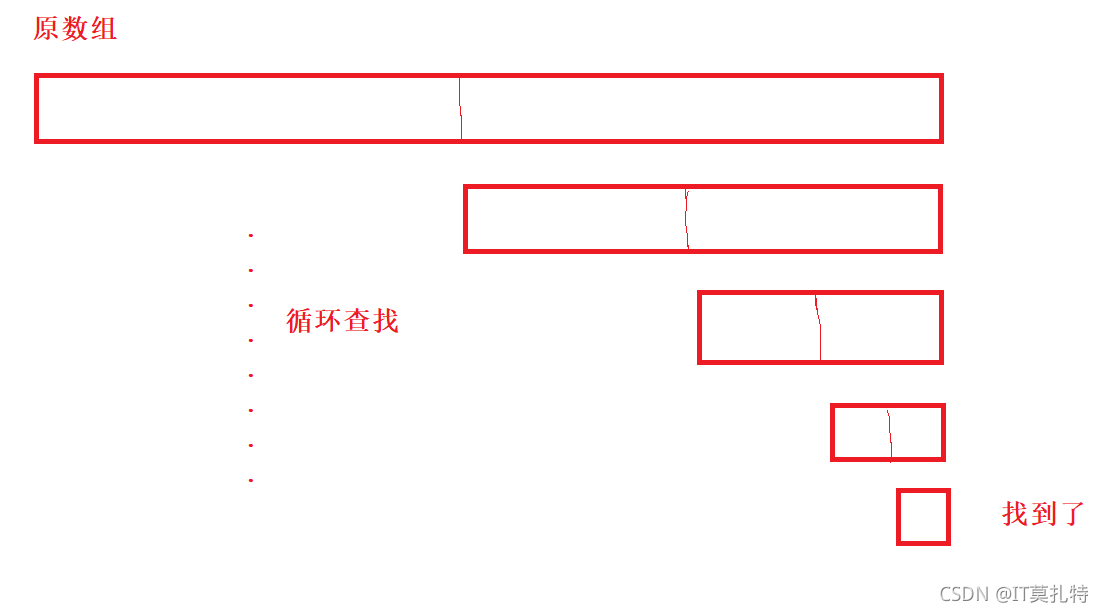最坏情况：O(log N)

每次确定中间下标，划分左右区间的时候都会除以2，那么
N / 2
N / 4
N / 8
.
.
.
1 //等于1的时候找到了
当找到了，要想知道它的执行次数的时候，通过它的展开就能知道
1 * 2 * 2 * 2 * 2 = N
2 ^ x = N
x = log N (以2为底，N的对数)

平均情况：不考虑
最好情况：O(1)

计算一下这个算法的时间复杂度

// 计算阶乘递归Factorial的时间复杂度？long long Factorial(size_t N) { 	return N < 2 ? N : Factorial(N-1)*N; }


时间复杂度：O(N)
这个算法，递归的开始条件是当N >= 2，它的结束条件是N < 2，当条件成立的时候会一直递归下去，直达条件为假递归终止才会将结果返回，递归的深度决定的是算法的时间复杂度，
N - 1
N - 2
N - 3
.
.
2
1 //递归结束，递归的层数是N

计算一下这个算法的时间复杂度

// 计算斐波那契递归Fibonacci的时间复杂度？long long Fibonacci(size_t N) { 	return N < 2 ? N : Fibonacci(N-1)+Fibonacci(N-2);}


时间复杂度：O(2 ^ N)
经典的递归分治算法，读者可以将它看成一个完全二叉树，在它递归层层展开的时候开辟的栈帧看作是一个结点，每创建一个结点都是一次函数调用，那么在脑海中想象出来的场景是这样的，可以看到它的调用次数是 Fib(N) = 2 ^0 + 2 ^1 + 2 ^ 2 + … + 2 ^(n -1) - x
时间复杂度：O(2 ^ N)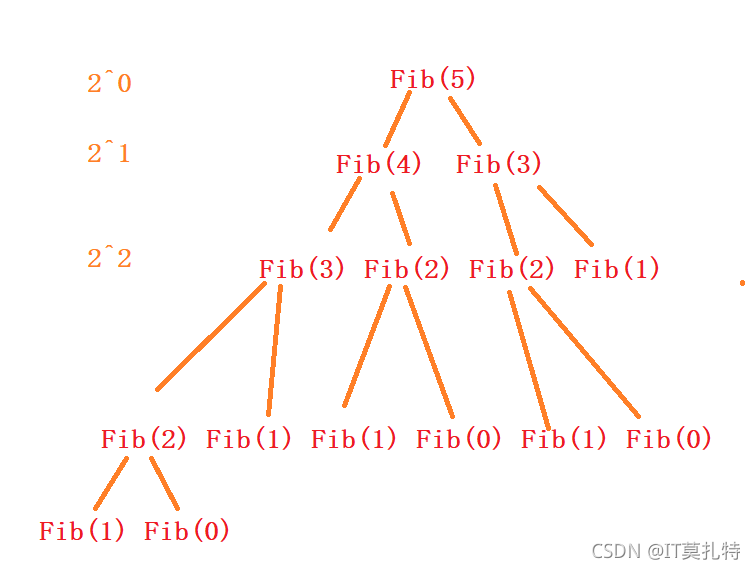计算一下这个算法的空间复杂度

// 计算BubbleSort的空间复杂度？void BubbleSort(int* a, int n) {  assert(a); for (size_t end = n; end > 0; --end) {  	int exchange = 0; 	for (size_t i = 1; i < end; ++i)	 { 	 	if (a[i-1] > a[i]) 		{  			Swap(&a[i-1], &a[i]); 			exchange = 1; 		} 	} 	 	if (exchange == 0) 		break; }}


空间复杂度：O(1)，常数个额外的变量

计算一下这个算法的空间复杂度

// 计算Fibonacci的空间复杂度？long long* Fibonacci(size_t n) {  if(n==0) return NULL;  long long * fibArray =(long long *)malloc((n+1) * sizeof(long long)); fibArray = 0; fibArray = 1;for (int i = 2; i <= n ; ++i) {  fibArray[i ] = fibArray[ i - 1] + fibArray [i - 2]; } return fibArray ;}


空间复杂度O(N)，malloc了N+1个long long大小的空间

计算一下这个算法的空间复杂度

long long Factorial(size_t N) { 	 return N < 2 ? N : Factorial(N-1)*N; }


空间复杂度O(N)，之前说时间复杂度的时候讲这个例子是每调用一次这个函数就会开辟一次栈帧，调用了N次

long long Fib(size_t N){ 	if(N < 3)		return	else		return Fib(N - 1) + Fib(N - 2); }


空间复杂度是O(N)
再之前讲时间复杂度的时候用这个例子，说的是将它看成一个完全二叉树，在它递归层层展开的时候开辟的栈帧看作是一个结点，每创建一个结点都是一次函数调用，当然计算时间复杂度符合这个特性，因为时间是不可复用的，而如果以空间复杂度的角度来计算的话，结果却并不是这样子，因为空间是可以复用的，每次递归调用，当Fib(N-1)递归完了，就会将内存归还给操作系统，进而才递归Fib(N - 2)，所以空间复杂度是O(N)

# 常见复杂度对比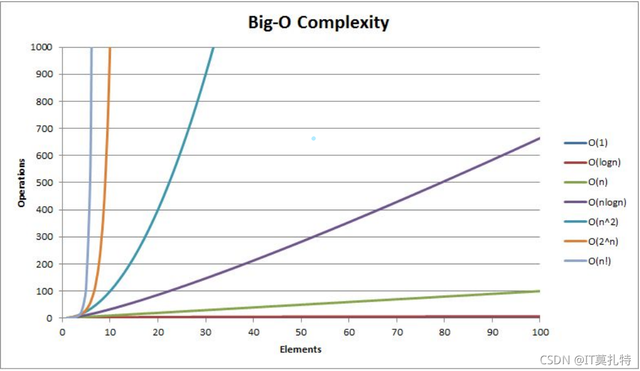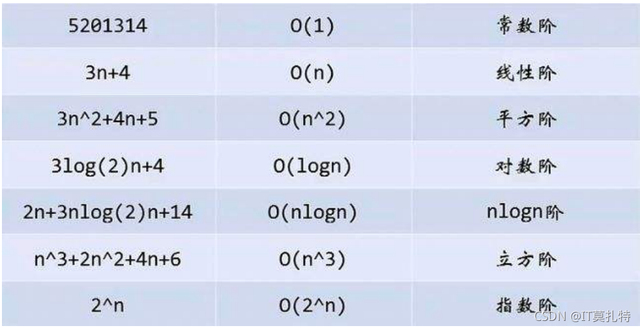# oj练习

17.04. 消失的数字

题目描述：
数组nums包含从0到n的所有整数，但其中缺了一个。请编写代码找出那个缺失的整数。你有办法在O(n)时间内完成吗？

• 思路一：快速排序 时间复杂度是O(N * log N)，并不符合题目要求
• 思路一：0 ~N个数字全部加起来再减去数组中的所有数字加起来，得到的结果就是缺失的那个数，时间复杂度O(N)
• 思路三：内存映射，将数组元素值出现的次数放在对应的一个数组下标位置，时间复杂度是O(N)
• 思路四：异或，将m与0 ~ numsize个数字和0 ~ numsize数组元素异或，最后再与数组长度异或，时间复杂度是O(N)
//实现思路四：int missingNumber(int* nums, int numsSize){     int m = 0;    int i = 0;    while(i < numsSize)    {         m ^= i;        m ^= nums[i++];    }        m ^= numsSize;    return m;}


旋转数组
题目描述：

给定一个数组，将数组中的元素向右移动 k 个位置，其中 k 是非负数实现思路：
数组元素整体逆置，【0，numsSize - 1】
左区间逆置，【0，k - 1】
右区间逆置，【k，numsize - 1】

//逆置数组void reverse(int* nums1,int *nums2){    while(nums1 < nums2)   {        int tmp = *nums1;       *nums1 = *nums2;       *nums2 = tmp;       ++nums1;       --nums2;   }   }void rotate(int* nums, int numsSize, int k){     if(k >= numsSize)    k %= numsSize;        reverse(nums, nums + numsSize - 1);    reverse(nums,nums + k - 1);    reverse(nums + k,nums + numsSize - 1);   }
整体逆置，【0，numsSize - 1】
> 左区间逆置，【0，k - 1】
> 右区间逆置，【k，numsize - 1】



//逆置数组void reverse(int* nums1,int *nums2){ while(nums1 < nums2) { int tmp = *nums1; *nums1 = *nums2; nums2 = tmp; ++nums1; --nums2; } }void rotate(int nums, int numsSize, int k){ if(k >= numsSize) k %= numsSize; reverse(nums, nums + numsSize - 1); reverse(nums,nums + k - 1); reverse(nums + k,nums + numsSize - 1); }

展开全文数据结构 空间复杂度
• ## 算法的时间复杂度和空间复杂度

千次阅读 多人点赞 2022-07-01 23:05:18
算法的时间复杂度和空间复杂度

# 1.算法效率

## 1.1 如何衡量一个算法的好坏

如何衡量一个算法的好坏呢？比如对于以下斐波那契数列：

long long Fib(int N)
{
if (N < 3)
return 1;
return Fib(N - 1) + Fib(N - 2);
}


斐波那契数列的递归实现方式非常简洁，但简洁一定好吗？那该如何衡量其好与坏呢？

## 1.2算法的复杂度

算法在编写成可执行程序后，运行时需要耗费时间资源和空间(内存)资源 。因此衡量一个算法的好坏，一般是从时间和空间两个维度来衡量的，即时间复杂度空间复杂度

时间复杂度主要衡量一个算法的运行快慢，而空间复杂度主要衡量一个算法运行所需要的额外空间。在计算机发展的早期，计算机的存储容量很小。所以对空间复杂度很是在乎。但是经过计算机行业的迅速发展，计算机的存储容量已经达到了很高的程度。所以我们如今已经不需要再特别关注一个算法的空间复杂度。

# 2.时间复杂度

## 2.1 时间复杂度的概念

时间复杂度的定义：在计算机科学中，算法的时间复杂度是一个函数，它定量描述了该算法的运行时间。一个算法执行所耗费的时间，从理论上说，是不能算出来的，只有你把你的程序放在机器上跑起来，才能知道。但是我们需要每个算法都上机测试吗？是可以都上机测试，但是这很麻烦，所以才有了时间复杂度这个分析方式。一个算法所花费的时间与其中语句的执行次数成正比例，算法中的基本操作的执行次数，为算法的时间复杂度

即：找到某条基本语句与问题规模N之间的数学表达式，就是算出了该算法的时间复杂度。

下面举个例子：

请计算一下Func1中++count语句总共执行了多少次？

void Func1(int N)
{
int count = 0;
for (int i = 0; i < N; ++i)
{
for (int j = 0; j < N; ++j)
{
++count;
}
}
for (int k = 0; k < 2 * N; ++k)
{
++count;
}
int M = 10;
while (M--)
{
++count;
}
printf("%d\n", count);
}


Func1 执行的基本操作次数 ： F(N) = N^2 + 2*N + 10

代入数字计算一下

N = 10 F(N) = 130
N = 100 F(N) = 10210
N = 1000 F(N) = 1002010

可以发现当N越来越大的时候，数字的大小主要取决于N^2了。

实际中我们计算时间复杂度时，我们其实并不一定要计算精确的执行次数，而只需要大概执行次数，那么这里我们使用大O的渐进表示法。

## 2.2 大O的渐进表示法

大O符号（Big O notation）：是用于描述函数渐进行为的数学符号。

推导大O阶方法：

1、用常数1取代运行时间中的所有加法常数。
2、在修改后的运行次数函数中，只保留最高阶项。
3、如果最高阶项存在且不是1，则去除与这个项相乘的常数。得到的结果就是大O阶。

使用大O的渐进表示法以后，Func1的时间复杂度为： O(N^2)

N = 10 F(N) = 100
N = 100 F(N) = 10000
N = 1000 F(N) = 1000000

通过上面我们会发现大O的渐进表示法去掉了那些对结果影响不大的项，简洁明了的表示出了执行次数。

另外有些算法的时间复杂度存在最好、平均和最坏情况：

最坏情况：任意输入规模的最大运行次数(上界)
平均情况：任意输入规模的期望运行次数
最好情况：任意输入规模的最小运行次数(下界)

例如：在一个长度为N数组中搜索一个数据x

最好情况：1次找到
最坏情况：N次找到
平均情况：N/2次找到

在实际中一般情况关注的是算法的最坏运行情况，所以数组中搜索数据时间复杂度为O(N)

## 2.3常见时间复杂度计算举例

实例1

计算Func2的时间复杂度

void Func2(int N)
{
int count = 0;
for (int k = 0; k < 2 * N; ++k)
{
++count;
}
int M = 10;
while (M--)
{
++count;
}
printf("%d\n", count);
}


基本操作执行了2*N + 10次，而通过推导大O阶方法

用常数1取代加法常数，得到2*N + 1

只保留最高阶项，得到2*N

将最高阶项的系数变为1，得到N

所以最后的时间复杂度是O(N)

实例2

计算Func3的时间复杂度

void Func3(int N, int M)
{
int count = 0;
for (int k = 0; k < M; ++k)
{
++count;
}
for (int k = 0; k < N; ++k)
{
++count;
}
printf("%d\n", count);
}


时间复杂度为O(N+M)

实例3

计算Func4的时间复杂度

void Func4(int N)
{
int count = 0;
for (int k = 0; k < 100; ++k)
{
++count;
}
printf("%d\n", count);
}


用常数1替代100，时间复杂度是O(1)

实例4

计算strchr的时间复杂度

const char* strchr(const char* str, int character)
{
while (*str != character)
{
str++;
}
return str;
}


最快执行了1次，最慢执行了N次，所以时间复杂度是O(N)

实例5

计算BubbleSort的时间复杂度

void BubbleSort(int* a, int n)
{
assert(a);
for (size_t end = n; end > 0; --end)
{
int exchange = 0;
for (size_t i = 1; i < end; ++i)
{
if (a[i - 1] > a[i])
{
Swap(&a[i - 1], &a[i]);
exchange = 1;
}
}
if (exchange == 0)
break;
}
}


第一趟冒泡排序了N - 1次，第二趟冒泡排序了N - 2次，依次类推，排序这个基本操作在最坏的情况下一共执行了(N*(N+1)/2次，而最好的情况下是数组已经排好了，此时只需要执行N次，时间复杂度取最坏的情况，所以是O(N^2)

实例6

计算BinarySearch的时间复杂度

int BinarySearch(int* a, int n, int x)
{
assert(a);
int begin = 0;
int end = n - 1;
// [begin, end]：begin和end是左闭右闭区间，因此有=号
while (begin <= end)
{
int mid = begin + ((end - begin) >> 1);
if (a[mid] < x)
begin = mid + 1;
else if (a[mid] > x)
end = mid - 1;
else
return mid;
}
return -1;
}


假如有序数组有N个数，那么查找一次就会将数组的范围缩小一半，直到最后只剩下一个数

可以这么用数字表示：

N / 2  / 2  / 2  / 2  / 2  / 2 ...... / 2  / 2  = 1

假设查找了x次，也就是每次将数组缩小一半(除以2)这个基本操作执行了x次，那么这个x与N之间的关系是2^x = N

那么x = logN，这里默认底数为2

所以时间复杂度是O(logN)

实例7

计算阶乘递归Fac的时间复杂度

long long Fac(size_t N)
{
if (0 == N)
return 1;
return Fac(N - 1) * N;
}


基本操作递归了N次，所以时间复杂度为O(N)

实例8

计算斐波那契递归Fib的时间复杂度

long long Fib(size_t N)
{
if (N < 3)
return 1;
return Fib(N - 1) + Fib(N - 2);
}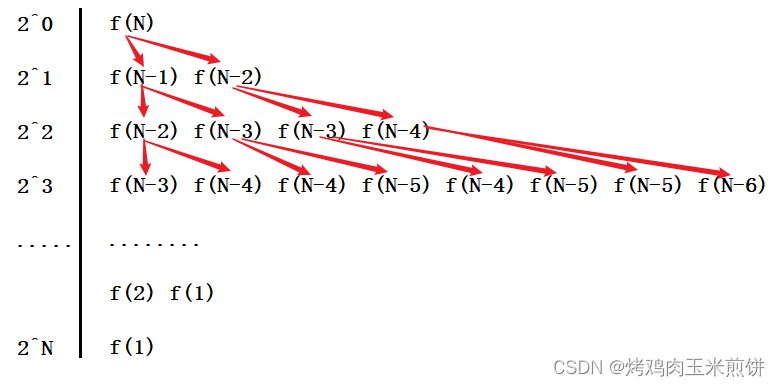基本操作递归了约为2^N次，根据推到大O阶的方法，所以最后的时间复杂度为O(N)

# 3.空间复杂度

空间复杂度也是一个数学表达式，是对一个算法在运行过程中临时占用存储空间大小的量度

空间复杂度不是程序占用了多少bytes的空间，因为这个也没太大意义，所以空间复杂度算的是变量的个数。空间复杂度计算规则基本跟实践复杂度类似，也使用大O渐进表示法

注意：函数运行时所需要的栈空间(存储参数、局部变量、一些寄存器信息等)在编译期间已经确定好了，因此空间复杂度主要通过函数在运行时候显式申请的额外空间来确定。

实例1

计算BubbleSort的空间复杂度

void BubbleSort(int* a, int n)
{
assert(a);
for (size_t end = n; end > 0; --end)
{
int exchange = 0;
for (size_t i = 1; i < end; ++i)
{
if (a[i - 1] > a[i])
{
Swap(&a[i - 1], &a[i]);
exchange = 1;
}
}
if (exchange == 0)
break;
}
}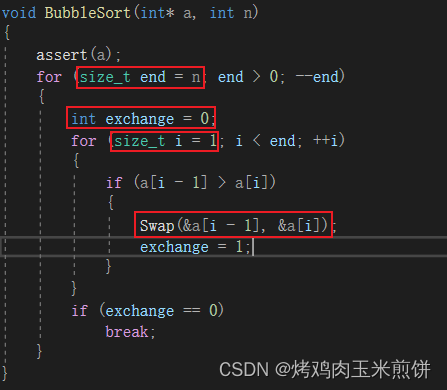可见，红框标注的地方，是在函数的内部额外创建了4个变量，也就是开辟了常数个额外空间，所以空间复杂度为O(1)

实例2

计算Fibonacci的空间复杂度

// 返回斐波那契数列的前n项
long long* Fibonacci(size_t n)
{
if (n == 0)
return NULL;
long long* fibArray = (long long*)malloc((n + 1) * sizeof(long long));
fibArray = 0;
fibArray = 1;
for (int i = 2; i <= n; ++i)
{
fibArray[i] = fibArray[i - 1] + fibArray[i - 2];
}
return fibArray;
}


在动态内存中开辟了N+1个sizeof(long long)大小的空间，所以空间复杂度为O(N)

实例3

计算阶乘递归Fac的空间复杂度

long long Fac(size_t N)
{
if (N == 0)
return 1;
return Fac(N - 1) * N;
}


递归调用了N次，开辟了N个栈帧，每个栈帧使用了常数个空间，所以空间复杂度为O(N)

实例4

计算Fibonacci的空间复杂度

long long Fib(size_t N)
{
if (N < 3)
return 1;
return Fib(N - 1) + Fib(N - 2);
}


每一次递归调用时，每两个子函数用的函数栈帧空间都是同一个，所以只额外开辟了N个栈帧，空间复杂度为O(N)

# 4.常见复杂度对比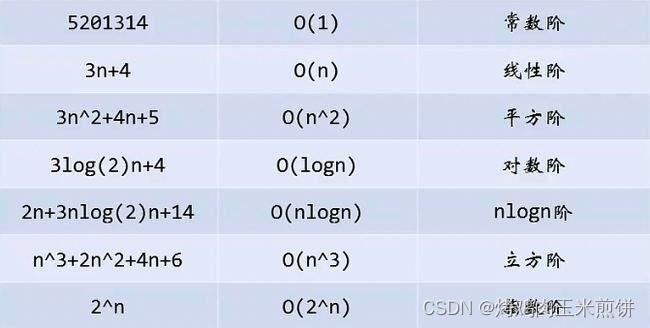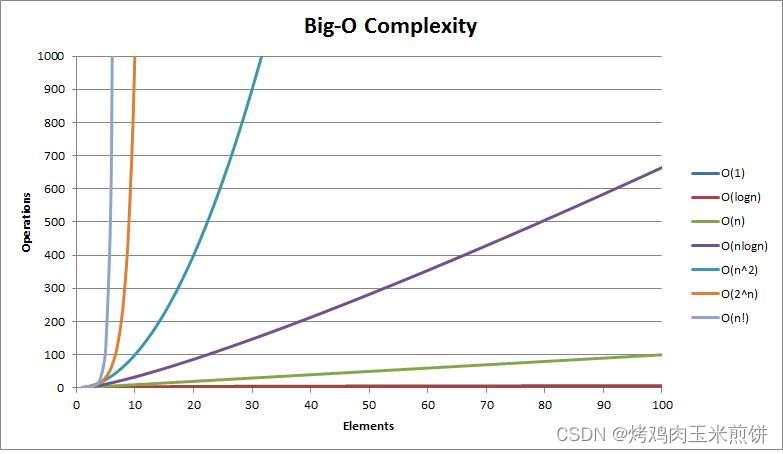# 5.复杂度的OJ练习

## 5.1消失的数字OJ链接：

https://leetcode-cn.com/problems/missing-number-lcci/

题目描述：

数组nums包含从0到n的所有整数，但其中缺了一个。请编写代码找出那个缺失的整数。你有办法在O(n)时间内完成吗？

示例 1：

输入：[3,0,1]
输出：2

示例 2：

输入：[9,6,4,2,3,5,7,0,1]
输出：8

这里给出时间复杂度都为O(N)的思路

1.创建一个大小为N + 1的数组，然后用-1将数组初始化，再将题目中给定数组中的数字放到新创建数组中对应下标的位置，最后将新数组中的数字遍历一遍，找出-1所对应的下标，该下标的数字就是所要找的消失的数字了。

2.将题目给定数组中的数字全都异或一次，再与从0到N+1的数字全部异或一次，就可以得到那个消失的数字了，其思路类似于在一个数组中寻找单身狗。

3.将题目给定数组进行快速排序，而后进行二分查找，找不到的那个数字即为要找的数字了。

4.将N+1个数字进行全都加起来，然后减去题目给定数组中的N个数字，最后得到的数字就是要找的消失的数字了。

## 3.2 旋转数组OJ链接：

https://leetcode-cn.com/problems/rotate-array/

题目描述：

给你一个数组，将数组中的元素向右轮转 k 个位置，其中 k 是非负数。

示例 1:

输入: nums = [1,2,3,4,5,6,7], k = 3
输出: [5,6,7,1,2,3,4]
解释:
向右轮转 1 步: [7,1,2,3,4,5,6]
向右轮转 2 步: [6,7,1,2,3,4,5]
向右轮转 3 步: [5,6,7,1,2,3,4]

示例 2:

输入：nums = [-1,-100,3,99], k = 2
输出：[3,99,-1,-100]
解释:
向右轮转 1 步: [99,-1,-100,3]
向右轮转 2 步: [3,99,-1,-100]

这里给出3种思路：

1.将最后一个数用一个临时变量保存起来，然后将数组中前面的数依次往后挪动，最后将临时变量中的数放到数组的第一个位置，这样的操作循环k次，最坏的情况下是k=N-1，这时时间复杂度是O(N^2)，而空间复杂度是O(1)，因为只开辟了1个临时变量，并且这个变量的空间是重复利用的。

2.额外开辟一个同样大小的数组，然后按照k的大小截取数据依次放入数组中，这种做法的时间复杂度为O(N)，空间复杂度为O(N)，这是以空间来换时间的做法。

3.根据k的大小将数组分位2个部分，第1个部分和第2个部分分别自旋，最后再将整个数组自旋一次，由于旋转交换的过程中只开辟了一个临时变量的空间，所以空间复杂度为O(1)，时间复杂度为O(N)。

void reverse(int* nums, int left, int right)
{
while (left < right)
{
int tmp = nums[left];
nums[left] = nums[right];
nums[right] = tmp;
++left;
--right;
}
}

void rotate(int* nums, int numsSize, int k){
k %= numsSize;
reverse(nums, 0, numsSize - 1 - k);
reverse(nums, numsSize - k, numsSize - 1);
reverse(nums, 0, numsSize - 1);
}

关于算法的时间复杂度和空间复杂度就先讲到这里了，今后也会不定期更新。

展开全文• 时间复杂度和空间复杂度，是用来评估算法的运行效率的，以此评估算法的优劣程度。 不需要特别准确的计算，是一个估算值。 一、时间复杂度 算法中的基本操作的执行次数，为算法的时间复杂度。 二、 三、 .....

时间复杂度和空间复杂度，是用来评估算法的运行效率的，以此评估算法的优劣程度。

不需要特别准确的计算，是一个估算值。

# 一、时间复杂度

算法中的基本操作的执行次数，为算法的时间复杂度。

PS：一般是看算法最坏的运行情况，也就是时间最长的情况。

计算时间复杂度，不需计算精确的执行次数，而只需要大概的执行次数。

大O的渐进表示法：

1. 用常数1取代运行时间中的所有加法常数。
2. 在修改后的运行次数函数中，只保留最高阶项。
3. 如果最高阶项存在且不是1，则去除与这个项目相乘的常数。得到的结果就是大O阶。

假设一个函数：

void fun1()
{
for(int i=0;i<10000;i++){
printf("O\n");
}
}

这里不管 i ，循环多少次。只要循环次数还是常数，其时间复杂度都是O(1)。

void fun2(int n)
{
for(int i=0;i<n;i++){
printf("O\n");
}
}

这种写法函数循环中执行次未知，取决于给与的函数参数N，所以可以说函数的时间复杂度为O(N)。

void fun3(int n,int m)
{
int count=0;
for(int k=0;k<m;k++){
++count;
}
for(int k=0;k<n;k++){
++count;
}
}

不知道N和M的大小，时间复杂度就是O(N+M)如果N远大于M，这里就可以认为其实O(N)M远大于N，这里就可以认为其为O(M)。

PS：虽然时间复杂度跟循环有联系，但是不能单纯数循环，主要还是看算法思想。

冒泡排序的时间复杂度：

void BubbleSort(int* a, int n)
{
for (size_t end = n; end > 0; --end)
{
int exchange = 0;
for (size_t i = 1; i < end; ++i)
{
if (a[i-1] > a[i])
{
Swap(&a[i-1], &a[i]);
exchange = 1;
}
}

if (exchange == 0)
break;
}
}

这里是两层循环嵌套，每次进入一个内部循环，都要执行 n-i 次。

也就是执行N-1，N-2，N-3，...，2，1。

是个等差数列，首项加尾项，乘以项数，除以2。

F(N)=(N-1+1)*(N-1)/2=N(N-1)/2； 有因为大O表示法只保留最高阶，去掉常数项得到冒泡排序的时间复杂度：O(N^2)；

二分查找时间复杂度：

int BinarySearch(int *a,int n,int x)
{
int begin=0;
int end=n-1;
while(begin<=end){
int mind=begin+((end-begin)>>1);
if(a[mid]<x){
begin=mid+1;
}
else if(a[mid]>x){
end=mid-1;
}
else{
return mid;
}
}
return -1;
}

思考算法最坏的情况，没有找的要查找的数字。每次查找的时候，没有找到，会一次性排除大概一半的数据(有奇数偶数的情况)。那么可以推导出执行的次数：

F(N)=1*2*2*2*2 ... *2=N；（1表示最后一次）

也可以 N/2/2/2.../2=1； 会发现除了多少个2，就说明语句执行了几次，2^x=N；就能得到时间复杂度：O(logN)；（log以2为底，文本不好表示，一般都表示2为底）

递归求阶乘的时间复杂度：

long long Fac(size_t N)
{
if(0==N)
return 1;
return Fac(n-1)*n;
}

代码执行次数为：

F(N)、F(N-1)、F(N-2)...、F(0)；每一次的时间复杂度是O(1)，会执行N+1次，常数忽略。得到时间复杂度：O(N)；

递归求菲波那切数列时间复杂度：

long long Fib(size_t N)
{
if(N<3)
return 1;
return Fib(N-1)+Fib(N-2);
}

虽然使用递归的写法代码间接明了，但是时间消耗非常高。

每次进入递归后，都会分成两个分支，一个n-1，一个n-2，

2^0       Fib(N)

2^1       Fib(N-1)                    Fib(N-2)

2^2       Fib(N-2)    Fib(N-3)   Fib(N-3)   Fib(N-4)
...
Fib(3)   Fib(2) ...
2^(N-2) Fib(2)   Fib(1) ...

每次调用都是O(N)，看调用多少次，最后一次调用是第 2^（N-2）次，全部加起来。

是等比数列：2^0+2^1+2^2+...2^(N-2) = 2^(N-1)-1；去掉常数项，得时间复杂度：O(2^N)；

PS：时间复杂度是成指数级增长的，所以递归计算斐波那契数列非常消耗时间。一般这种时间复杂度的函数是不会被使用的。

循环求斐波那契数列时间复杂度：

long long*Fibonacci(size_t n)
{
if(n==0)
return NULL;

long long * FibArray(long long*)malloc((n+1)*sizeof(long long));

FibArray=0;
FibArray=1;

for(int i=2;i<=n;++i){
FibArray[i]=FibArray[i-1]+FibArray[i-2];
}

return FibArray;
}

会发现只需要循环执行N次就可以计算出结果，其时间复杂度为：O(N)。

程序执行1024次

时间复杂度实际执行次数
冒泡排序：O(N^2)1,048,576次
二分查找：O(logN) 10次

会发现使用时间复杂度就能直接比较函数的优劣了，这里明显二分查找比冒泡排序效率高。

# 二、空间复杂度

对一个算法在运行过程中临时占用存储空间大小的量度

实际上因为现在硬件的不断提高，所以现在不怎么考虑空间复杂度 ，大多都是使用空间换时间。

冒泡排序的空间复杂度：

void BubbleSort(int* a, int n)
{
for (size_t end = n; end > 0; --end)
{
int exchange = 0;
for (size_t i = 1; i < end; ++i)
{
if (a[i-1] > a[i])
{
Swap(&a[i-1], &a[i]);
exchange = 1;
}
}

if (exchange == 0)
break;
}
}

会发现冒泡函数没有申请额外的空间，使用的是参数传入的指定空间，所以函数的空间复杂度为：O(1)；

循环求斐波那契数列空间复杂度：

long long*Fibonacci(size_t n)
{
if(n==0)
return NULL;

long long * FibArray(long long*)malloc((n+1)*sizeof(long long));

FibArray=0;
FibArray=1;

for(int i=2;i<=n;++i){
FibArray[i]=FibArray[i-1]+FibArray[i-2];
}

return FibArray;
}

计算空间复杂度看其额外生成的空间，函数使用malloc申请了n个大小的空间，所以空间复杂度为：O(N)；

递归求阶乘的空间复杂度：

long long Fac(size_t N)
{
if(0==N)
return 1;
return Fac(n-1)*n;
}

函数每次进入递归，都会申请一个堆栈空间。

Fac(N)，Fac(N-1)，Fac(N-2)，...，Fac(0)，一共建立了N+1个栈帧，所以递归求阶乘的空间复杂度为：O(N)；

PS：虽然空间复杂度不高，但是因为栈区是比较小的，一旦栈帧建立太多会照成栈溢出。

其实绝大多数的算法，空间复杂度不是O(N)就是O(1)。

如果在递归函数中加上，

int*p=(int *)malloc(sizeof(int) *N)；

每次进入递归都都会申请空间，这样空间复杂度会变成：O(N^2)；

递归求斐波那契数列空间复杂度：

long long Fib(size_t N)
{
if(N<3)
return 1;
return Fib(N-1)+Fib(N-2);
}

2^0       Fib(N)

2^1       Fib(N-1)                    Fib(N-2)

2^2       Fib(N-2)    Fib(N-3)   Fib(N-3)   Fib(N-4)
...
Fib(3)   Fib(2) ...
2^(N-2) Fib(2)   Fib(1) ...

递归求斐波那契数列时间消耗非常高，但是空间开销小。递归调用每次进递归建立一个栈帧，出递归销毁一个栈帧，然后继续进行递归时会重复利用已经销毁过的栈帧。所以递归使用的空间，是最深的一次递归线路的空间。

这里最深的线路是最前面的一条线，执行了 N-2次，所以函数的空间复杂度为：O(N)；

PS：时间累计。空间不累计，可以重复利用。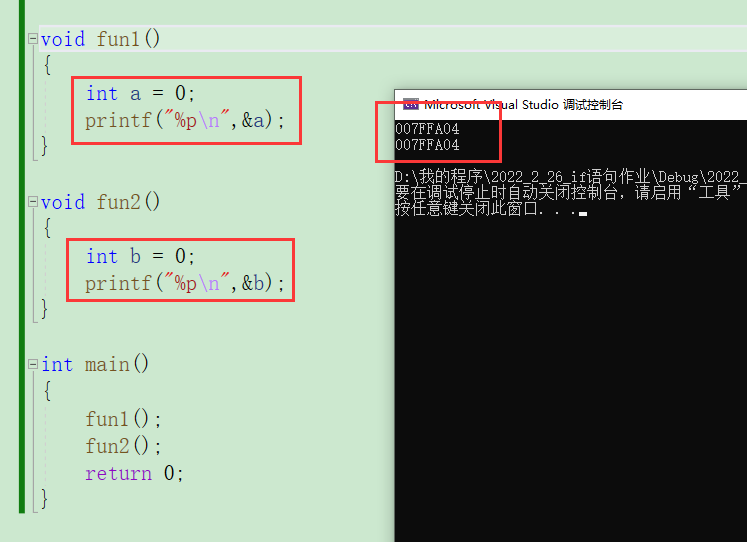会发现两个地址是一样的，说明的确栈区空间是重复利用的。

# 总结：

在很多的算法题目中，都要求控制时间复杂度和空间复杂度，要充分了解和知晓其特点。

展开全文• 想要学好算法，必须要掌握如何分析一个算法的时间复杂度和空间复杂度，只有自己会分析这两个个衡量算法主要性能的标准，才能更好的写出性能优秀的算法，复杂度同时也可以分为最好时间复杂度，最坏时间复杂度，平均...数据结构 空间复杂度 平均时间复杂度
• 对java的8种排序方法的空间复杂度和时间复杂度，进行了一个简单的统计java
• 如何度量一个算法的执行效率/时间呢？...叫做算法的渐进时间复杂度，简称时间复杂度。大O表示算法的执行时间T（n）与f（n）成正比。例如：在一个数组中，找到值等于X的数据。我们需要遍历数组，依次每个元素数据结构 排序算法 空间复杂度
• ## 简析时间复杂度和空间复杂度

万次阅读 多人点赞 2019-06-25 16:24:17
时间复杂度和空间复杂度是用来评价算法效率高低的2个标准，身为开发者肯定会经常会听到这2个概念，但它们分别是什么意思呢? 其实这两个概念从字面意思上也能看出一二： 时间复杂度：就是说执行算法需要消耗的时间...空间复杂度
• 文章目录1.算法效率2.时间复杂度大O渐近表示法3.空间复杂度 1.算法效率 算法效率分析分为两种：第一种是时间效率，第二种是...计算时间复杂度和空间复杂度时，为了估算一个算法的耗时情况，不需要计算出精确的执行次java
• ## 时间复杂度和空间复杂度计算

多人点赞 热门讨论 2022-04-18 15:12:33数据结构
• 时间复杂度和空间复杂度是考量算法性能的重要指标，尤其是时间复杂度，因为计算时间的快慢，直接影响到用户的体验，而占用的内存可以在物理形式上解决。 先说说什么是算法？ 算法是解决编程问题的代码实现过程，比如...空间复杂度 大O表示法
• 时间维度事后统计法事前分析估算的方法时间复杂度(1)时间频度(2)时间复杂度大O符号表示法常见的时间复杂度量级常数阶O(1)线性阶O(n)对数阶O(logN)线性对数阶O(nlogN)平方阶O(n^2^)立方阶O(n³)、K次方阶O(n^k)二、...
• 订阅更多算法小知识1.1时间复杂度O(1)代码只被执行一次O(n)for循环里的代码执行了n次O(1)+O(n)= O(n)当n足够大 ，1可以忽略不计O(n)*O(n)= O(n^2)如果存在嵌套就相乘O(logN)用于求2的多少次方为N1.2空间复杂度一个...数据结构 空间复杂度
• 时间效率被称为时间复杂度，而空间效率被称作空间复杂度时间复杂度主要衡量的是一个算法的运行速度，而空间复杂度主要衡量一个算法所需要的额外空间，在计算机发展的早期，计算机的存储容量很小。所以对空间...java
• 1.什么是时间复杂度和空间复杂度 1.1时间复杂度 1.2空间复杂度 2.如何计算时间复杂度和空间复杂度 2.1使用大O阶方法 2.2计算一些常用算法的时间复杂度 2.3计算一些常用算法的空间复杂度 3.对复杂度有要求的...
• 时间复杂度衡量一个算法的运行快慢，而空间复杂度衡量一个算法所需要的额外空间（但由摩尔定律（每18个月计算机内存增加一倍）的存在，空间复杂度不再需要特别被关注）。 二、时间复杂度总结的六点 算法的时间...数据结构
• 时间复杂度和空间复杂度的概念及各种算法的时间复杂度 及举例 算法的复杂度可分为俩种 一种时间复杂度 另一种是空间复杂度。 俩者的概念：时间复杂度是指执行这个算法所需要的计算工作量；而空间复杂度是指执行这个...
• 2，时间复杂度 时间频度：一个算法花费的时间与算法中语句的执行次数成正比例，哪个算法中语句的执行次数多，它花费的时间就多。一个算法中语句的执行次数称为语句频度或者时间频度，记为T(n)。在数量及运算下，...数据结构 排序算法 空间复杂度
• 时间复杂度和空间复杂度 如何计算？推导算法：大O推导法时间复杂度定义常数阶线性阶对数阶平方阶小结空间复杂度定义 推导算法：大O推导法 1、用常数1取代运行时间中的所有加法常数 2、在修改后的运行次数函数中，只...空间复杂度
• 排序算法-算法时间复杂度和空间复杂度概念 详细讲解 排序算法的介绍 排序也称排序算法(Sort Algorithm)，排序是将一组数据，依指定的顺序进行排列的过程。 排序的分类： 1)内部排序： 指将需要处理的所有数据都加载...java...